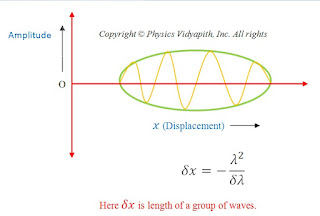### Definition and derivation of the phase velocity and group velocity of wave

Wave:
A wave is defined as a disturbance in a medium from an equilibrium condition that propagates from one region of the medium to other regions.

When such type of wave propagates in the medium a progressive change in phase takes place from one particle to the next particle.

Propagation of Wave: Wave propagation in the medium occurs with two different kinds of velocity. i.e. phase velocity and group velocity.

1. Phase Velocity:
The velocity with which plane of constant the phase of a wave propagates through the medium at a certain frequency is called the phase velocity or wave velocity.

A plane wave traveling in the positive x-direction is represented by

$y=A sin \omega (t-\frac{x}{v})$

Where $\omega$ – angular frequency

$y=A sin(\omega t-\frac{\omega x}{v})$

$y=A sin(\omega t-kt) \qquad(1)$

For plane-wave $(\omega t-kx)$ is the phase of wave motion. For the plane of constant phase (wavefront). We have

$( \omega t-kx) = constant (\phi) \qquad(2)$

Differentiate with respect to time $(t)$ of the above equation

$\omega -k.\frac{dx}{dt}=0$

$\frac{dx}{dt}=\frac{ \omega}{k}\qquad(3)$

$V_{p}=\frac{ \omega}{k} \qquad \left [ \because V_{p}= \frac{dx}{dt} \right ]$

Where $V_{p}$ - Phase Velocity of a wave

Question- Show that the phase velocity of matter-wave always exceeds the velocity of light.

$V_{p}=\frac{ \omega }{k}$

$V_{p}=\frac{2\pi \nu }{\frac{2\pi }{\lambda }}$

$V_{p} = \frac{2 \pi h \nu}{\frac{2 \pi h}{\lambda }}$

$V_{P} = \frac{E}{P}$

$V_{P} =\frac{mc^{2}}{mv}$

$V_{P} =\frac{c^{2}}{v}$

Method-II:

$V_{P}=\nu \lambda$

$V_{P}=\frac{mc^{2}}{h} \frac{h}{mv}$

$V_{P}=\frac{c^{2}}{v}$

Where $v$ is the velocity of matter particles.

Wave packet→
A wave packet is an envelope or packet which contains the number of plane waves having different wavelengths or wavenumbers. These numbers of waves superimpose on each other and form constructive and destructive interference over a small region of space and a resultant wave obtain. The spread of amplitude of the resultant wave with distance determines the size of the wave packet. A wave packet is also called a wave group.Group Velocity→
The velocity of propagation of wave packet through space is known as group velocity.

Group Velocity also represents the velocity of energy flow or transmission of information in a traveling wave or wave packet. It is represented by $V_{g}$. So

$V_{g} = \frac{d\omega }{dk}$

Expression for group velocity $(V_{g})$→

Let two plane simple harmonic waves of the same amplitude and slightly different wavelength traveling simultaneously in the positive x-direction in a dispersive medium be represented by –

$y_{1} = A\: sin{(\omega t-kx)} \qquad (1)$

$y_{2}= A\:sin[(\omega +\delta \omega )t-(k+\delta k)x] \qquad (2)$

From the superposition principle the resultant displacement of waves

$y=y_{1}+y_{2}\qquad (3)$

$y=A \: sin(\omega t-kx)+ A \: sin[(\omega +\delta \omega)t-(k+\delta k)x]$

$y=A[sin(\omega t-kx)+ sin{(\omega +\delta \omega )t-(k+\delta k )x}]$

$y=2Asin(\omega t-kx)cos\frac{1}{2}(t\delta \omega –x\delta k) \qquad (4)$

This is the analytical equation for the group of waves. This equation represents the following points –
1. The sine factors represent a carrier wave that travels with the phase velocity.

2. The amplitude of the resultant wave is

$R=2A cos \frac{1}{2}(t \delta\omega-x \delta k) \qquad (5)$

(A) For maximum amplitude

$cos\frac{1}{2}(\delta\omega t-x\delta k)=1 \qquad (6)$

Then the resultant amplitude of the wave packet will be

$R_{m}=2A \qquad$ {from equation $(5)$ }

Where $R_{m}$ - Resultant maximum amplitude

From equation$(6)$-

$cos\frac{1}{2}(t\delta\omega –x\delta k)=1$

$cos\frac{1}{2}(t\delta\omega –x\delta k)=cos0$

$\frac{1}{2}(t\delta\omega –x\delta k)=0$

$\frac{x}{t}=\frac{\delta\omega }{\delta k}$

$V_{g}=\frac{\delta\omega }{\delta k}$

OR

$V_{g}=\frac{d\omega}{dk}$

Thus maximum amplitude i.e. the center of the wave packet moves with velocity $\frac {d\omega}{dk}$ or group velocity.

(B) For minimum amplitude

Let a wave packet that has minimum (zero) amplitude on two successive points $x_{1}and x_{2}$. So for minimum amplitude at point $x_{1}$ –

$cos\frac{1}{2}(t.\delta \omega –x_{1}.\delta k)=0$

$cos\frac{1}{2}(t.\delta \omega –x_{1}.\delta k)=cos(2n+1)\frac{\pi }{2}$

$\frac{1}{2}(t.\delta \omega –x_{1}.\delta k)=(2n+1)\frac{\pi }{2}$

$t.\delta \omega – x_{1}.\delta k=(2n+1)\pi \qquad(1)$

Similarly minimum amplitude at the second successive point $x_{2}$-

$t\delta \omega –x_{2}.\delta k =(2n-1)\pi \qquad(2)$

Now subtract the equation $(2)$ in equation $(1)$

$(t.\delta \omega – x_{1}.\delta k) – (t.\delta \omega - x_{2}.\delta k)= (2n+1)\pi–(2n-1)\pi$

$(x_{2}-x_{1}).\delta k=2\pi$

$x_{2}-x_{1}=\frac{2\pi}{\delta k}$

$x_{2}-x_{1}=\frac{2\pi}{d(\frac{2\pi}{\lambda})}$

$\delta x=\frac{-\lambda ^{2}}{\delta \lambda }$

Here $\delta x$ -Length of the wave packet

The above equation also can be written as-

$dx=\frac{-\lambda^{2}}{d \lambda }$

Prove that

$V_{g}= -\lambda^{2} \frac{d\nu }{d\lambda}$

Where $\nu$ is the frequency and $\lambda$ is the wavelength.

Proof:

We know that

$V_{g}=\frac{d\omega }{dk}$

$V_{g}=\frac{d(2\pi\nu )}{d(\frac{2\pi }{\lambda })} \qquad \left( \because \omega= 2\pi \nu \: and \: k=\frac{2\pi}{\lambda } \right)$

$V_{g}=\frac{2\pi d\nu }{2\pi d( \frac{1}{ \lambda })}$

$V_{g}=-\lambda ^{2}\frac{d\nu }{d\lambda }$

Note: A moving particle cannot be equal to a single wave train. The speed of a single wave train is called the phase velocity so moving particles are equivalent to a group of waves or wave packets.